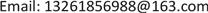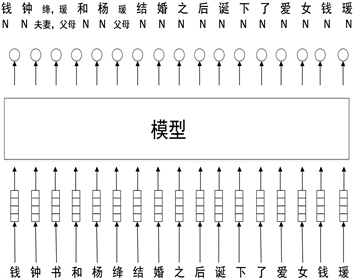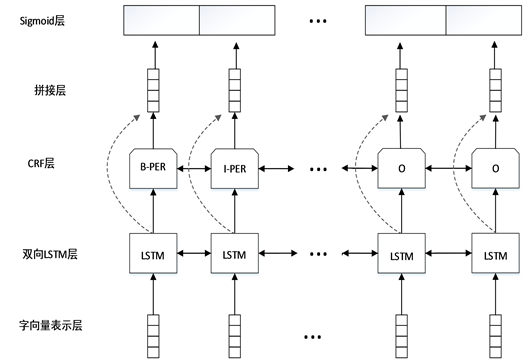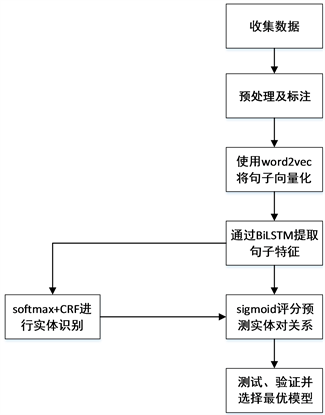﻿ 面向中文的端到端多实体关系提取研究 End-to-End Multi-Entity Relationship Extraction for Chinese Text

Computer Science and Application
Vol. 09  No. 12 ( 2019 ), Article ID: 33293 , 10 pages
10.12677/CSA.2019.912251

End-to-End Multi-Entity Relationship Extraction for Chinese Text

Hao Yang

Institute of Network Technology, Beijing University of Posts and Telecommunications, BeijingReceived: Nov. 14th, 2019; accepted: Nov. 29th, 2019; published: Dec. 5th, 2019ABSTRACT

Relationship extraction can obtain key information in the text. Entity relationship extraction is a method to identify entities in unstructured text and extract the relationships between entity pairs. For the traditional relationship extraction with the help of external NLP tools and local classification, the end-to-end entity relationship extraction model can reduce the error propagation between pipeline models and obtain better results. An end-to-end entity relationship extraction model based on BiLSTM was designed to solve the problem of multiple entity pairs in Chinese texts by making decisions on the possibility of corresponding entities and relationships. In Chinese text, three kinds of entity recognition and 10 kinds of relation extraction are realized. The experimental results show that this method can achieve a better result of multi-entity relation extraction without manual construction of complex features.

Keywords:Entity Relation Extraction, End-to-End, BiLSTM, Multiple Entity Pairs1. 引言Figure 1. Input and output of sentences

2. 相关研究

3. 模型设计Figure 2. End-to-end entity relation extraction model structureFigure 3. Working flow chart of the model

3.1. 数据预处理

3.2. 字向量表示层

3.3. BiLSTM编码层

LSTM模型能够很好的捕获较长距离的依赖。LSTM主要包括细胞状态，临时细胞状态，隐层状态，遗忘门，记忆门和输出门。LSTM主要通过对细胞状态中信息遗忘和记忆新的信息来进行信息的传递，有用的信息保留，无用的信息遗忘。LSTM模型适合对时序数据的建模。但是LSTM无法编码从后到前的信息，所以采用BiLSTM可以更好的编码上下文信息。LSTM模型的运行细节如下公式所示：

${f}_{t}=\sigma \left({W}_{f}\cdot \left[{h}_{t},{x}_{t}\right]+{b}_{f}\right)$ (1)

${i}_{t}=\sigma {\left({W}_{i}\cdot \left[{h}_{t-1},{x}_{t}\right]+b\right)}_{i}$ (2)

${\stackrel{˜}{C}}_{t}=\mathrm{tanh}\left({W}_{C}\cdot \left[{h}_{t-1},{x}_{t}\right]+{b}_{C}\right)$ (3)

${C}_{t}={f}_{t}*{C}_{t-1}+{i}_{t}*\stackrel{˜}{C}$ (4)

${o}_{t}=\sigma \left({W}_{o}\cdot \left[{h}_{t-1},{x}_{t}\right]+{b}_{o}\right)$ (5)

${h}_{t}={o}_{t}*\mathrm{tanh}\left({C}_{t}\right)$ (6)

3.4. 实体识别层

$S\left({w}_{1}^{\left(e\right)},\cdots ,{w}_{n}^{\left(e\right)}\right)=\underset{i=0}{\overset{n}{\sum }}{s}_{i,{w}_{i}^{\left(e\right)}}^{e}+\underset{i=1}{\overset{n-1}{\sum }}{T}_{{w}_{i}^{\left(e\right)},{w}_{i+1}^{\left(e\right)}}$ (7)

CRF得分主要由两部分组成，一部分是LSTM预测标签得分，另一部分是表示从一个标签到另一个标签的CRF转移矩阵得分，用softmax分类器计算各个实体标签的概率，得到交叉熵损失函数Lner ，通过最小化损失函数优化实体识别模型的参数。

3.5. 关系提取层

${s}^{\left(r\right)}\left({w}_{i},{w}_{j},{r}_{k}\right)={V}^{\left(r\right)}f\left({U}^{\left(r\right)}{w}_{i}+{W}^{\left(r\right)}{w}_{j}+{b}^{\left(r\right)}\right)$ (8)

$P\left(head\text{=}{w}_{i},label\text{=}{r}_{k}|{w}_{j}\right)\text{=}\sigma \left({s}^{\left(r\right)}\left({w}_{i}\text{,}{w}_{j},{r}_{k}\right)\right)$ (9)

${L}_{rc}=\underset{i=0}{\overset{n}{\sum }}\underset{j=0}{\overset{m}{\sum }}-\mathrm{log}P\left(head={w}_{i,j},rel={r}_{i,j}|{w}_{i}\right)$ (10)

m是关系标签集中的关系数量。经过训练后，让实体wi 对应的实体wj和关系标签rk的估计联合概率超过阈值，我们可以预测多个关系对。对于端到端实体关系提取任务，我们计算的目标函数是Lner + Lrc

4. 实验

4.1. 实验环境和评价指标

$P\text{=}\frac{正确识别的实体对关系数}{识别的实体对关系数}×\text{100%}$ (11)

$R\text{=}\frac{正确识别的实体对关系数}{样本实体对关系数}×\text{100%}$ (12)

$F=\frac{2×P×R}{P+R}×100%$ (13)

4.2. 实验数据

4.3. 实验设计

4.4. 实验结果及分析

$s\left(W\right)={L}_{ner}\left(W\right)×{L}_{rc}\left(W\right)$ (14)

5. 结语

End-to-End Multi-Entity Relationship Extraction for Chinese Text[J]. 计算机科学与应用, 2019, 09(12): 2256-2265. https://doi.org/10.12677/CSA.2019.912251

1. 1. 王超. 基于深度学习的中文微博人物关系图谱的研究与实现[D]: [硕士学位论文]. 武汉: 武汉邮电科学研究院, 2018.

2. 2. 唐敏. 基于深度学习的中文实体关系抽取方法研究[D]: [硕士学位论文]. 成都: 西南交通大学, 2018.

3. 3. 谢星辰. 互联网人物属性识别与融合方法研究[D]: [硕士学位论文]. 成都: 电子科技大学, 2018.

4. 4. 黄蓓静. 深度学习技术在中文人物关系抽取中的应用研究[D]: [硕士学位论文]. 上海: 华东师范大学, 2017.

5. 5. Mintz, M., Bills, S., Snow, R. and Jurafsky, D. (2009) Distant Supervision for Relation Extraction without Labeled Data. Proceedings of the Joint Conference of the 47th Annual Meeting of the ACL and the 4th International Joint Conference on Natural Language Processing of the AFNLP, Singapore, 2-7 August 2009, 1003-1011. https://doi.org/10.3115/1690219.1690287

6. 6. Culotta, A., McCallum, A. and Betz, J. (2006) Integrating Probabil-istic Extraction Models and Data Mining to Discover Relations and Patterns in Text. Proceedings of the Main Conference on Human Language Technology Conference of the North American Chapter of the Association of Computational Lin-guistics, New York, 4-9 June 2006, 296-303. https://doi.org/10.3115/1220835.1220873

7. 7. Doddington, G.R., Mitchell, A., Przybocki, M.A., et al. (2004) The Automatic Content Extraction (ACE) Program-Tasks, Data, and Evaluation. Proceedings of the Fourth International Conference on Language Resources and Evaluation, Lisbon, Portugal.

8. 8. Zelenko, D., Aone, C. and Richardella, A. (2003) Kernel Methods for Relation Extraction. Journal of Machine Learning Research, 3, 1083-1106.

9. 9. Gábor, K., Buscaldi, D., Schumann, A.K., et al. (2018) Semeval-2018 Task 7: Semantic Relation Extraction and Classification in Scientific Papers. Proceedings of The 12th International Workshop on Semantic Evaluation, New Orleans, LA, 679-688. https://doi.org/10.18653/v1/S18-1111

10. 10. Zhang, M., Zhang, Y. and Fu, G. (2017) End-to-End Neural Relation Extraction with Global Optimization. Proceedings of the 2017 Conference on Empirical Methods in Natural Language Processing, Copenhagen, Denmark, 1730-1740. https://doi.org/10.18653/v1/D17-1182

11. 11. Ammar, W., Peters, M., Bhagavatula, C., et al. (2017) The AI2 System at SemEval-2017 Task 10 (ScienceIE): Semi-Supervised End-to-End Entity and Relation Extraction. Proceedings of the 11th International Workshop on Semantic Evaluation (SemEval-2017), Vancouver, 592-596. https://doi.org/10.18653/v1/S17-2097

12. 12. Ren, X., Wu, Z., He, W., et al. (2017) CoType: Joint Extraction of Typed Entities and Relations with Knowledge Bases. Proceedings of the 26th International Confer-ence on World Wide Web, Perth, Australia, 3-7 April 2017, 1015-1024. https://doi.org/10.1145/3038912.3052708

13. 13. Rink, B. and Harabagiu, S. (2010) Utd: Classifying Semantic Rela-tions by Combining Lexical and Semantic Resources. Proceedings of the 5th International Workshop on Semantic Evalu-ation, Los Angeles, CA, 15-16 July 2010, 256-259.

14. 14. Tu, C., Zhang, Z., Liu, Z. and Sun, M. (2017) TransNet: Translation-Based Network Representation Learning for Social Relation Extraction. Proceedings of International Joint Conference on Artificial Intelligence (IJCAI), Melbourne, 2864-2870. https://doi.org/10.24963/ijcai.2017/399

15. 15. 司文豪, 贾雷萍, 戚银城. 基于卷积神经网络的中文人物关系抽取方法[J]. 计算机与现代化, 2018(9): 17-20.

16. 16. Santos, C.N., Xiang, B. and Zhou, B. (2015) Classifying Relations by Ranking with Convolutional Neural Networks. arXiv preprint arXiv:1504.06580.

17. 17. Nguyen, T.H. and Grishman, R. (2015) Relation Extraction: Perspec-tive from Convolutional Neural Networks. Proceedings of the 1st Workshop on Vector Space Modeling for Natural Language Processing, Denver, Co, 39-48. https://doi.org/10.3115/v1/W15-1506

18. 18. Meng, L. and Wu, B. (2017) Core Discovery and Relation Extraction in Organization Profiling. 2017 13th International Conference on Semantics, Knowledge and Grids, Beijing, 13-14 August 2017, 219-222. https://doi.org/10.1109/SKG.2017.00045

19. 19. Rotsztejn, J., Hollenstein, N. and Zhang, C. (2018) ETH-DS3Lab at SemEval-2018 Task 7: Effectively Combining Recurrent and Convolutional Neural Networks for Relation Classification and Extraction. arXiv Preprint arXiv:1804.02042. https://doi.org/10.18653/v1/S18-1112

20. 20. Peng, N., Poon, H., Quirk, C., Toutanova, K. and Yih, W.-T. (2017) Cross-Sentence N-Ary Relation Extraction with Graph LSTMS. arXiv Preprint arXiv:1708.03743. https://doi.org/10.1162/tacl_a_00049

21. 21. Zhang, D.X. and Wang, D. (2015) Relation Classification via Recurrent Neural Network. arXiv Preprint arXiv:1508.01006.

22. 22. Zhou, P., Shi, W., Tian, J., et al. (2016) Attention-Based Bidirectional Long Short-Term Memory Networks for Relation Classification. Proceedings of the 54th Annual Meeting of the Association for Computational Linguistics, 2, 207-212. https://doi.org/10.18653/v1/P16-2034

23. 23. Miwa, M. and Bansal, M. (2016) End-to-End Relation Extraction Using LSTMS on Sequences and Tree Structures. arXiv Preprint arXiv:1601.00770. https://doi.org/10.18653/v1/P16-1105

24. 24. Zheng, S., Hao, Y., Lu, D., et al. (2017) Joint Entity and Relation Ex-traction Based on a Hybrid Neural Network. Neurocomputing, 257, 59-66. https://doi.org/10.1016/j.neucom.2016.12.075